# 1. Sigmoid激活函数¶

• 函数表达式：$f(x)=\frac{1}{1+e^{-x}}$
• 函数图像：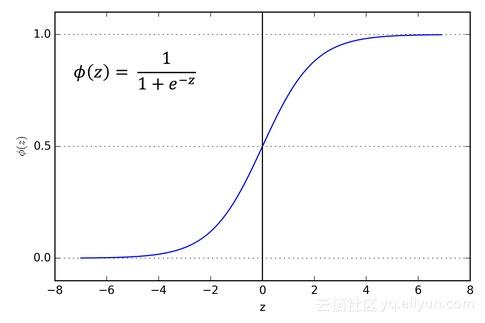• 导数：$f'(x)=f(x)(1-f(x))$
• 优点：Sigmoid激活函数是应用范围最广的一类激活函数，具有指数形状，它在物理意义上最为接近生物神经元。另外，Sigmoid的输出是(0,1)，具有很好的性质，可以被表示为概率或者用于输入的归一化等。可以看出，Sigmoid函数连续，光滑，严格单调，以(0,0.5)中心对称，是一个非常良好的阈值函数。当x趋近负无穷时，y趋近于0x趋近于正无穷时，y趋近于1x=0时，y=0.5。当然，在x超出[-6,6]的范围后，函数值基本上没有变化，值非常接近，在应用中一般不考虑。Sigmoid函数的导数是其本身的函数，即f′(x)=f(x)(1−f(x))，计算非常方便，也非常节省计算时间。
• 缺点：Sigmoid最明显的缺点就是饱和性。从曲线图中看到，其两侧的导数逐渐趋近于0，即：$\lim_{x->\infty}f'(x)0$ 。我们将具有这种性质的激活函数叫作软饱和激活函数。具体的，饱和又可分为左饱和与右饱和。与软饱和对应的是硬饱和, 即f′(x)=0，当|x|>c，其中c为常数。sigmoid 的软饱和性，使得深度神经网络在二三十年里一直难以有效的训练，是阻碍神经网络发展的重要原因。另外，Sigmoid函数的输出均大于0，使得输出不是0均值，这称为偏移现象，这会导致后一层的神经元将得到上一层输出的非0均值的信号作为输入。

# 2. TanH¶

• 函数表达式：$f(x)=\frac{1-e^{-2x}}{1+e^{-2x}}$
• 函数图像：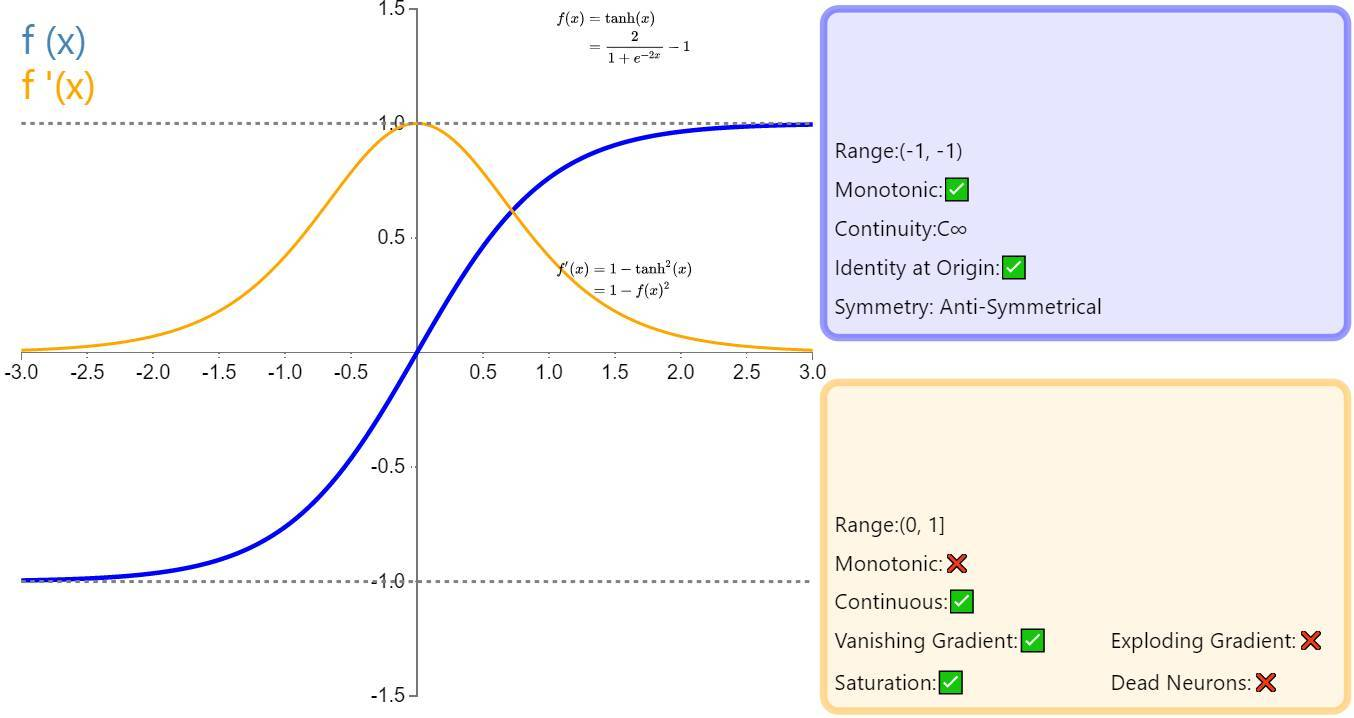• 导数：$f'(x)=1-f(x)^2$
• 优点：与Sigmoid相比，它的输出均值是0，使得其收敛速度要比Sigmoid快，减少迭代次数。
• 缺点：该导数在正负饱和区的梯度都会接近于0值(仍然具有软饱和性)，会造成梯度消失。还有其更复杂的幂运算。

# 3. ReLU¶

• 函数表达式：$f(x)=max(0,x)$
• 函数图像：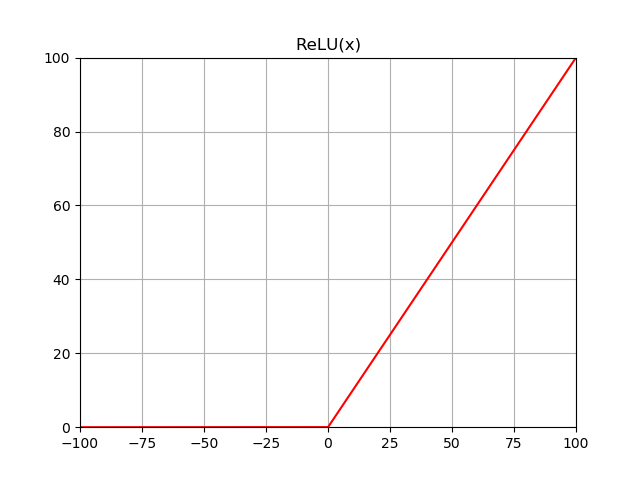• 导数：当$x>0$$f'(x)=1$，当$x<0$$f'(x)=0$
• 优点：ReLU的全称是Rectified Linear Units，是一种AlexNet时期才出现的激活函数。 可以看到，当x<0时，ReLU硬饱和，而当x>0时，则不存在饱和问题。所以，ReLU 能够在x>0时保持梯度不衰减，从而缓解梯度消失问题。这让我们能够直接以监督的方式训练深度神经网络，而无需依赖无监督的逐层预训练。
• 缺点：随着训练的推进，部分输入会落入硬饱和区，导致对应权重无法更新。这种现象被称为“神经元死亡”。与Sigmoid类似，ReLU的输出均值也大于0，偏移现象和神经元死亡会共同影响网络的收敛性。

# 4. Leaky ReLU & PReLU¶

• 函数表达式和导数：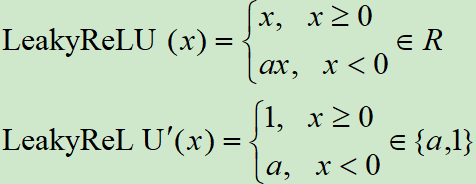• 函数图像：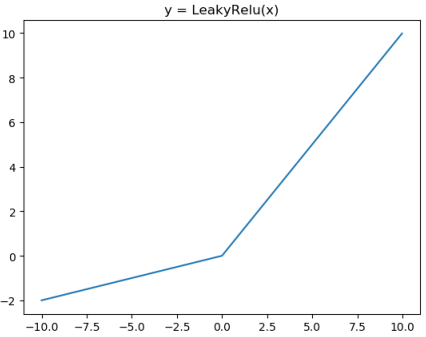• 特点：为了改善ReLU在$x<0$时梯度为$0$造成Dead ReLU，提出了Leaky ReLU使得这一问题得到了缓解。例如在我们耳熟能详的YOLOV3网络中就使用了Leaky ReLU这一激活函数，一般$a$$0.25$。另外PReLU就是将Leaky ReLU公式里面的$a$当成可学习参数参与到网络训练中。

# 5. ReLU6¶

• 函数表达式：$f(x)=min(max(0,x),6)$
• 特点：ReLU6就是普通的ReLU但是限制最大输出值为6（对输出值做clip），这是为了在移动端设备float16的低精度的时候，也能有很好的数值分辨率，如果对ReLU的激活范围不加限制，输出范围为0到正无穷，如果激活值非常大，分布在一个很大的范围内，则低精度的float16无法很好地精确描述如此大范围的数值，带来精度损失。

# 6. ELU¶

• 函数表达式：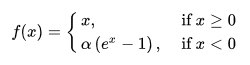• 函数图像：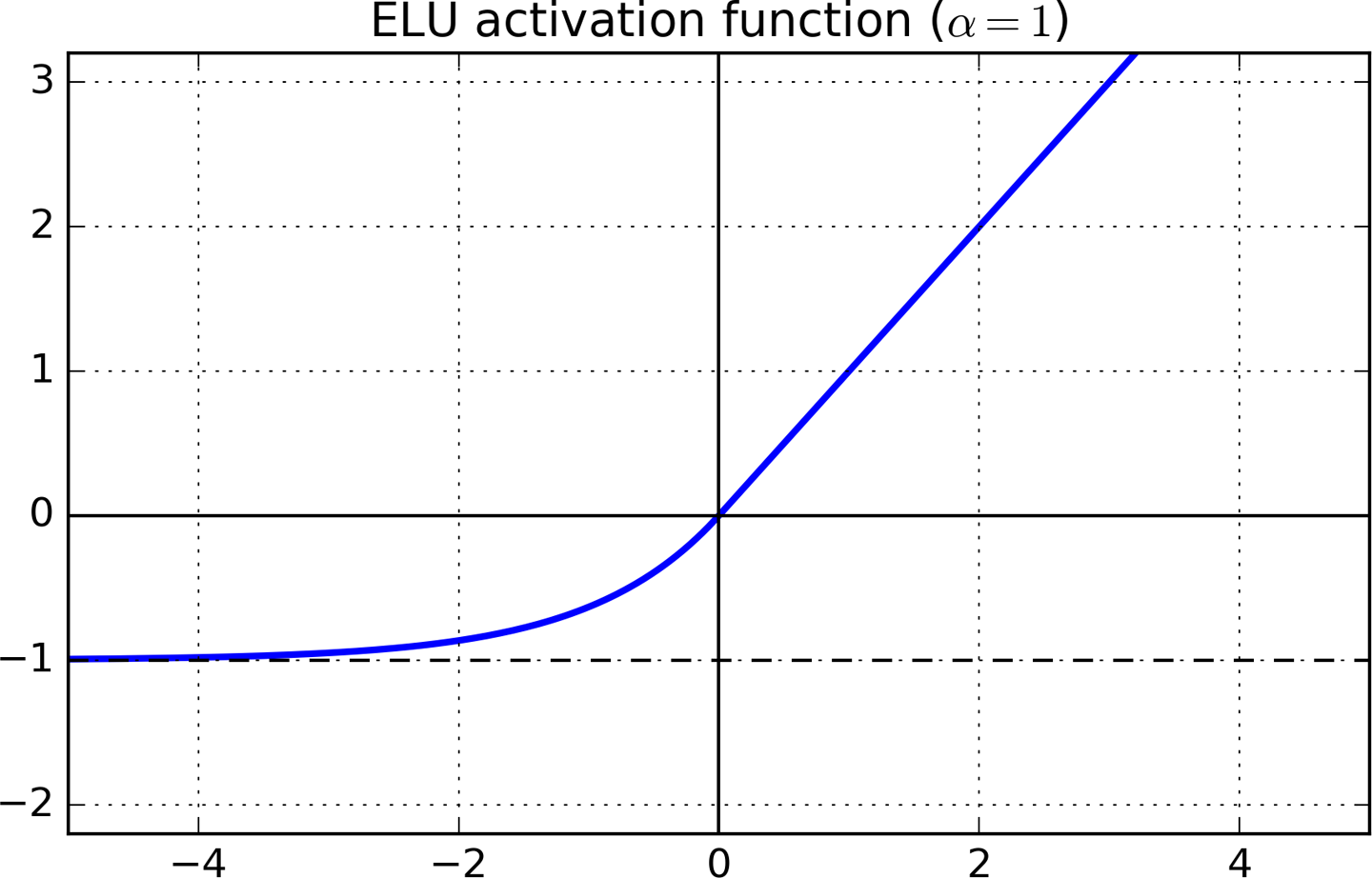• 导数：当$x>=0$时，$f'(x)=1$，当$x<0$时，$f'(x)=\alpha e^x$
• 特点：融合了sigmoid和ReLU，左侧具有软饱和性，右侧无饱和性。右侧线性部分使得ELU能够缓解梯度消失，而左侧软饱能够让ELU对输入变化或噪声更鲁棒。ELU的输出均值接近于零，所以收敛速度更快。在 ImageNet上，不加Batch Normalization 30层以上的ReLU网络会无法收敛，PReLU网络在MSRA的Fan-in （caffe ）初始化下会发散，而 ELU 网络在Fan-in/Fan-out下都能收敛。关于Fan-in/Fan-out初始化请看附录的参考2链接。

# 7. SoftSign¶

• 函数表达式：$f(x)=\frac{x}{1+|x|}$
• 函数图像：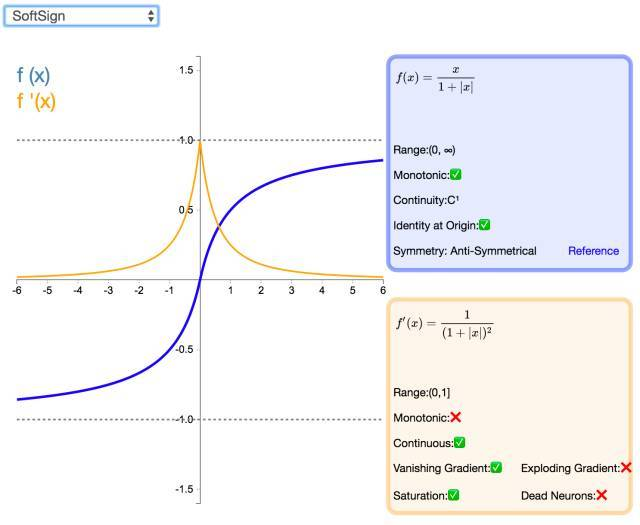• 导数：图中已经求出。
• 特点：Softsign是tanh激活函数的另一个替代选择，从图中可以看到它和tanh的曲线极其相似，不过相比于tanh，Softsign的曲线更平坦，导数下降的更慢一点，这个特性使得它可以缓解梯度消失问题，可以更高效的学习。

# 8. SoftPlus¶

• 函数表达式：$f(x)=ln(1+e^x)$
• 函数图像：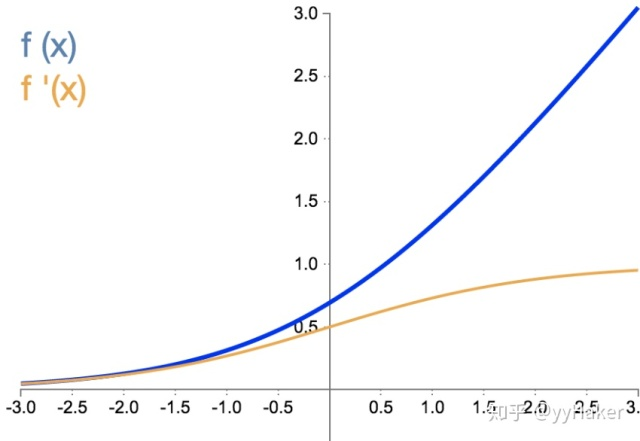• 函数导数：SoftPlus激活函数的导数恰好就是sigmoid激活函数，即$f'(x)=sigmoid(x)$
• 优点：SoftPlus可以作为ReLu的一个不错的替代选择，可以看到与ReLU不同的是，SoftPlus的导数是连续的、非零的、无处不在的，这一特性可以防止出现ReLU中的“神经元死亡”现象。
• 缺点：SoftPlus是不对称的，不以0为中心，存在偏移现象；而且，由于其导数常常小于1，也可能会出现梯度消失的问题。

# 9. SELU¶

• 函数表达式：$SELU(x)=\lambda * ELU(x)$，也即是：

$f(x)=\lambda \begin{cases} \alpha(e^x-1) & x \le 0 \\ x & x>0 \end{cases}$

• 特点：这个激活函数来自论文：https://arxiv.org/abs/1706.02515 。而这篇论文就是提出了这一激活函数，然后论文写了93页公式来证明只需要把激活函数换成SELU就能使得输入在经过一定层数之后变成固定的分布。。而这个函数实际上就是在ELU激活函数的基础上乘以了一个$\lambda$，但需要注意的是这个$\lambda$是大于1的。
• 更详细的讨论可以见这里：https://www.zhihu.com/question/60910412

# 10. Swish¶

• 函数表达式：$f(x)=x*sigmoid(x)$，其中$\beta$是个常数或可训练的参数.Swish 具备无上界有下界、平滑、非单调的特性。
• 函数图像：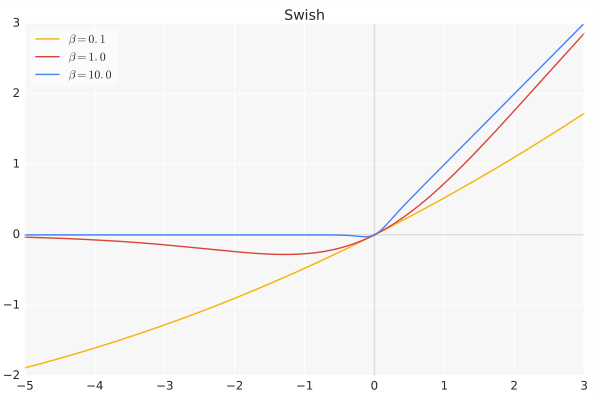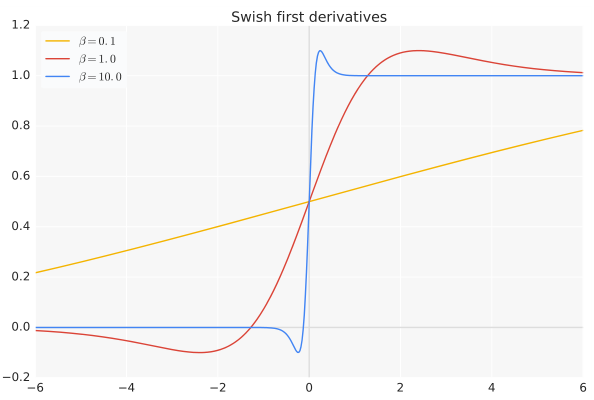• 特点：Swish 在深层模型上的效果优于 ReLU。例如，仅仅使用 Swish 单元替换 ReLU 就能把 Mobile NASNetA 在 ImageNet 上的 top-1 分类准确率提高 0.9%，Inception-ResNet-v的分类准确率提高 0.6%。当$\beta=0$时，Swish激活函数变成线性函数$f(x)=\frac{x}{2}$.而当$\beta->\infty$时，$\delta(x)=(1+exp(-x))^{-1}$为0或1,这个时候Swish激活函数变成ReLU激活函数$f(x)=2max(0,x)$。因此Swish激活函数可以看做是介于线性函数与ReLU函数之间的平滑函数。

# 11. Maxout¶

• 函数表达式：$f(x)=max(w_1^Tx+b_1,w_2^Tx+b_2,...,w_n^Tx+b_n)$
• 特点：Maxout模型实际上也是一种新型的激活函数，在前馈式神经网络中，Maxout的输出即取该层的最大值，在卷积神经网络中，一个Maxout特征图可以是由多个特征图取最值得到。 Maxout的拟合能力是非常强的，它可以拟合任意的的凸函数。但是它和Dropout一样需要人为设定一个$k$值。 为了便于理解，假设有一个在第$i$层有$2$个节点$i+1$层有$1$个节点构成的神经网络。即：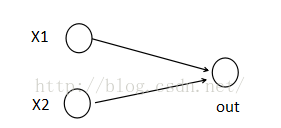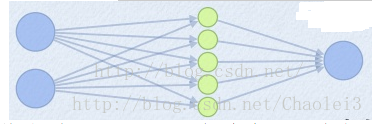$z1 = W1*X+b1$ $z2 = W2*X+b2$ $z3 = W3.X+b3$ $z4 = W4.X+b4$ $z5 = W4.X+b5$ $out = max(z1,z2,z3,z4,z5)$

# 12. Mish¶

• 函数表达式：$Mish(x)=x*tanh(ln(1+e^x))$
• 函数图像：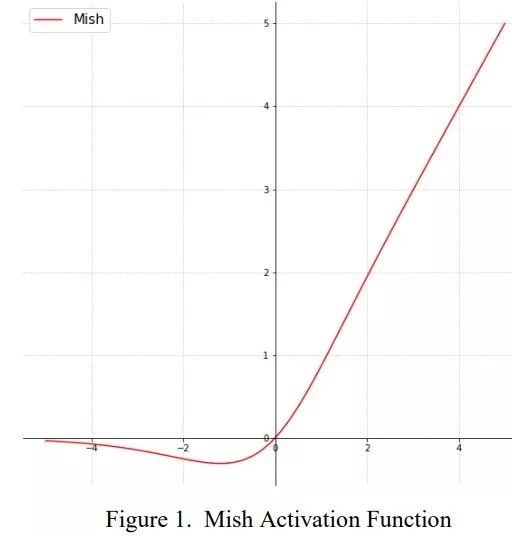• 特点：这个激活函数是最新的SOTA激活函数，我还没具体使用和了解过这个激活函数，就暂时不总结这一个了。不过论文中提到，以上无边界(即正值可以达到任何高度)避免了由于封顶而导致的饱和，理论上对负值的轻微允许更好的梯度流，而不是像ReLU中那样的硬零边界，并且整个损失函数仍然保持了平滑性。
• 论文名为：Mish: A Self Regularized Non-Monotonic Neural Activation Function

# 激活函数尝试经验¶

• 首先使用ReLU，速度最快，然后观察模型的表现。
• 如果ReLU效果不是很好，可以尝试Leaky ReLU或Maxout等变种。
• 尝试tanh正切函数(以零点为中心,零点处梯度为1)。
• 在深度不是特别深的CNN中，激活函数的影响一般不会太大。
• Kaggle比赛，试试Mish？

# 推荐阅读¶